Next: Heat and Work Up: Statistical Mechanics Previous: Behavior of Density of

# Exercises

1. Consider a particle of mass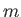confined within a cubic box of dimensions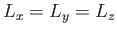. According to elementary quantum mechanics, the possible energy levels of this particle are given by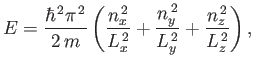where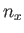,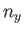, and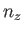are positive integers. (See Section C.10.)
1. Suppose that the particle is in a given state specified by particular values of the three quantum numbers,,,. By considering how the energy of this state must change when the length,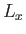, of the box parallel to the-axis is very slowly changed by a small amount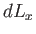, show that the force exerted by a particle in this state on a wall perpendicular to the-axis is given by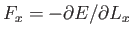.
2. Explicitly calculate the force per unit area (or pressure) acting on this wall. By averaging over all possible states, find an expression for the mean pressure on this wall. (Hint: exploit the fact that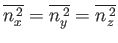must all be equal, by symmetry.) Show that this mean pressure can be written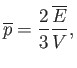where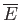is the mean energy of the particle, and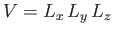the volume of the box.

2. The state of a system with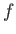degrees of freedom at time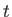is specified by its generalized coordinates,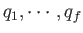, and conjugate momenta,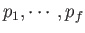. These evolve according to Hamilton's equations (see Section B.9):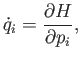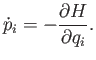Here,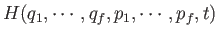is the Hamiltonian of the system. Consider a statistical ensemble of such systems. Let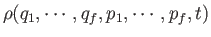be the number density of systems in phase-space. In other words, let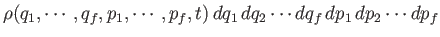be the number of states with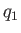lying betweenand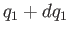,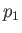lying betweenand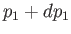, et cetera, at time.
1. Show that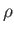evolves in time according to Liouville's theorem:[Hint: Consider how the the flux of systems into a small volume of phase-space causes the number of systems in the volume to change in time.]

2. By definition,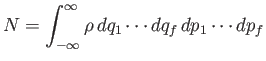is the total number of systems in the ensemble. The integral is over all of phase-space. Show that Liouville's theorem conserves the total number of systems (i.e.,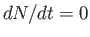). You may assume thatbecomes negligibly small if any of its arguments (i.e.,and) becomes very large. This is equivalent to assuming that all of the systems are localized to some region of phase-space.

3. Suppose that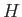has no explicit time dependence (i.e.,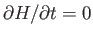). Show that the ensemble-averaged energy,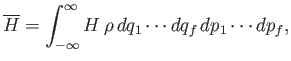is a constant of the motion.

4. Show that ifis also not an explicit function of the coordinate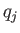then the ensemble average of the conjugate momentum,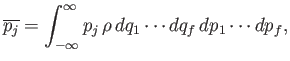is a constant of the motion.

3. Consider a system consisting of very many particles. Suppose that an observation of a macroscopic variable,, can result in any one of a great many closely-spaced values,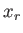. Let the (approximately constant) spacing between adjacent values be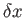. The probability of occurrence of the valueis denoted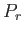. The probabilities are assumed to be properly normalized, so that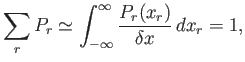where the summation is over all possible values. Suppose that we know the mean and the variance of, so that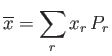and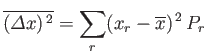are both fixed. According to the-theorem, the system will naturally evolve towards a final equilibrium state in which the quantity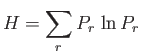is minimized. Used the method of Lagrange multipliers to minimixewith respect to the, subject to the constraints that the probabilities remain properly normalized, and that the mean and variance ofremain constant. (See Section B.6.) Show that the most general form for thewhich can achieve this goal is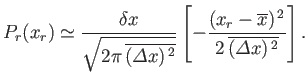This result demonstrates that the system will naturally evolve towards a final equilibrium state in which all of its macroscopic variables have Gaussian probability distributions, which is in accordance with the central limit theorem. (See Section 2.10.)Next: Heat and Work Up: Statistical Mechanics Previous: Behavior of Density of
Richard Fitzpatrick 2016-01-25